# Simple Linear Regression Tutorial for Machine Learning (ML)

## Diving into calculating a simple linear regression and linear best fit with code examples in Python and math in detail

Author(s): Pratik Shukla, Roberto Iriondo

Last updated, January 8, 2021

This tutorial’s code is available on Github and its full implementation as well on Google Colab.

📚 Check out our gradient descent tutorial. 📚

# What is a Simple Linear Regression?

Simple linear regression is a statistical approach that allows us to study and summarize the relationship between two continuous quantitative variables. Simple linear regression is used in machine learning models, mathematics, statistical modeling, forecasting epidemics, and other quantitative fields.

Out of the two variables, one variable is called the dependent variable, and the other variable is called the independent variable. Our goal is to predict the dependent variable’s value based on the value of the independent variable. A simple linear regression aims to find the best relationship between X (independent variable) and Y (dependent variable).

There are three types of relationships. The kind of relationship where we can predict the output variable using its function is called a deterministic relationship. In random relationships, there are no relationships between the variables. In our statistical world, it is not likely to have a deterministic relationship. In statistics, we generally have a relationship that is not so perfect, that is called a statistical relationship, which is a mixture of deterministic and random relationships .

## Examples:

1. Deterministic Relationship:

b. Fahrenheit = 1.8*celsius+32

2. Statistical Relationship:

a. Number of chocolates vs. cost

b. Income vs. expenditure

## Understanding Simple Linear Regression:

The simplest type of regression model in machine learning is a simple linear regression. First of all, we need to know why we are going to study it. To understand it better, why don’t we start with a story of some friends that lived in “Bikini Bottom” (referencing SpongeBob) .

SpongeBob, Patrick, Squidward, and Gary lived in the “Bikini Bottom!”. One day Squidward went to SpongeBob, and they had this conversation. Let’s check it out.

Squidward: “Hey, SpongeBob, I have heard that you are so smart!”

SpongeBob: “Yes, sir! There is no doubt in that.”

Squidward: “Is that so?”

SpongeBob: “Umm…Yes!”

Squidward: “So here is the thing. I want to sell my house as I am going to shift to my new lavish house downtown. But I cannot figure out at which price I should sell my house! If I keep the price too high, then no one will buy it, and if I set the price low, I might face a large financial loss! So you have to help me find the best price for my house. But, please keep in mind that you have only one day!”

SpongeBob stressed as always, but optimistic about finding the solution. To discuss the problem, he went to his wise friend Patrick’s house. Patrick is in his living room watching TV with a big bowl of popcorn in his hands, and after SpongeBob described the whole situation to Patrick:

Patrick: “That is a piece of cake. Follow me!”

(They decided to go to Squidward’s neighborhood, where his two neighbors recently sold their houses. After making some discreet inquiries, they obtained the following details from Squidward’s new neighbors. Now Patrick explained the whole plan to SpongeBob.)

Patrick: Once we have some essential data on how the previous house sells from Squidward’s neighborhood, I think we can make some logical deductions to predict Squidward’s house’s price. So let us get some data.

From the collected data, Patrick was able to plot the data on a scatter plot:

If we closely observe the graph above, we can notice that we can connect our two data points with a line, and as we know, each line has its equation. From figure 3, we can quickly get the house price if we have the house’s area. It will be easier for us if we get the house price by using some formula. Please note that we can get the house price by plotting a horizontal and vertical line on the graph, but to generalize it, we use the line equation. First, we need to see some basics of geometry and dive into the equation of the line.

## Basics of Coordinate Geometry:

1. We always look from left to right in the coordinate plane to name the points.
2. After looking from left-to-right, the first point we get must be named (x1,y1), and the second point will be (x2,y2).
3. Horizontal lines have a slope of 0.
4. Vertical lines have an “infinite” slope.
5. If the second point’s Y-coordinate is greater than the Y-coordinate of the first point, then the line has a positive(+) slope. The line has a negative slope.
6. Points at the same vertical distance from X-axis have the same Y-coordinate.
7. Points at the same vertical distance from Y-axis have the same X-coordinate.

Now let’s get back to our graph.

We all know that the equation of the line:

From the definition of the slope of a straight line:

From the rules mentioned above, we can infer that in our graph:

(X1 , Y1) = ( 1500 , 150000)

(X2 , Y2) = (2500 , 300000)

Next, we can easily find the slope of the two points.

Taking our example into consideration, in our equation, Y represents the house’s price, and X represents the area of the house.

Now since we have all the other values, we can calculate the value of slope b.

Notice that we can use any of the points to calculate the slope value. The answer to the slope will always be the same for the same straight line.
Next, since we have all our parameters, we can write the equation of line as:

To find the price of Squidward’s house, we need to plug-in X=1800 in the above equation.

Now, we can say that Squidward should sell his house for \$ 195,000.00. That was easy.

Please note that we only had two data points to quickly plot a single straight line through them and get our equation of a line. In this case, the critical thing to notice here is that our prediction will depend on the value of two data points. If we change the value of any of the two available data points, our prediction will likely also change. To cope with this problem, we have data sets in larger quantities. Real-world datasets may contain millions of data points.

Now let us get back to our example. When we have more than two data points in our dataset(the usual case), we cannot draw a single straight line that passes through all points, right? That is why we will use a line that best fits our data set. This line is called the best fit line or the regression line. By using this line’s equation, we will make predictions about our dataset.

Please note that the central concept remains the same. We will find the equation of the line and plug-in X’s value (independent variable) to find Y’s value (dependent variable). We need to find the best fit line for our dataset.

# Calculating the Linear Best Fit

As we can see in figure 11, we cannot plot a single straight line that passes through all the points. So what we can do here is to minimize the error. It means that we find a bar and then find the prediction error. Since we have the actual value here, we can easily find the error in prediction. Our ultimate goal will be to find the line that has the minimal error. That line is called the linear best fit.

As discussed above, our goal is to find the linear best fit for our dataset, or in other words, we can say that our goal should be to reduce the error in prediction. Now the question is, how do we calculate the error? One way to measure the distance between the scattered points and the line is to find the distance between their Y values.

To understand it better, let us get back to our actual house price prediction example. We know that the actual selling price of a house with an area of 1800 square feet is \$220,000. If we predict the house price based on the line equation, which is Y = 150X-75000, we will get the house price at \$ 195,000. Now here we can see that there is a prediction error.

Therefore, we can use the Sum of Squared error calculation technique to find the error in prediction for each of the data points. We randomly choose the parameters of our line and then calculate the error. Afterward, we will adjust the parameter again and then calculate the error.

We will repeat this until we get the minimum possible error. This process is a part of the gradient descent algorithm, which we will cover in later tutorials. We think now it is clear that we will recalculate the line’s parameters until we get the best fit line, or we get a minimum error in our prediction.

## 1. Positive error:

Actual selling price: \$ 220,000

Predicted selling price: \$ 195,000

Error in prediction: \$ 220,000–\$ 195,000 = \$25,000

## 2. Negative error:

Actual selling price: \$ 160,000

Predicted selling price: \$ 195,000

Error in prediction: \$160,000 — \$195,000 = -\$35,000

As we can see, it is also possible to get a negative error. To account for negative errors, we square the error.

To account for the negative values, we will square the errors.

Next, we have to find the parameters of a line that has the least error. Once we have that, we can form an equation of a line and predict the dataset’s data values. We will go through this part later in this tutorial.

## Guidelines for regression line:

1. Use regression lines when there is a significant correlation to predict values.
2. Stay within the range of the data, and make sure not to extrapolate. For example, if the data is from 10 to 60, do not try to predict a value for 500.
3. Do not make predictions for a population that base on another population’s regression line.

## Use-cases for linear regression:

1. Height and weight.
2. Alcohol consumption and blood alcohol content.
3. Vital lung capacity and pack-years of smoking.
4. The driving speed and gas mileage.

# Finding the Equation for the Linear Best Fit

Before we dive deeper into a simple linear regression formula’s derivation, we will try to find the best fit line parameters without using any formulas. Consider the following table with data points X and Y. The next table Y’ is the predicted value and Y-Y’ gives us the prediction error.

Next, we are going to use the sum of squares method to calculate the error. For such, we will have to find (Y-Y’)². Please note that we have three terms in each row of (Y — Y’). First, we will dive into the formula to find the square with three terms.

In our case, the value of (Y — Y’)² for each row will be:

Next, notice that we need to add all the squared terms in our formula of the error sum of squares.

Next, our goal is to determine the values of slope(m) and y-intercept(b). To find out the values, we will use the formula of the vertex in a second-degree polynomial.

Next, we need to rearrange our central equation to bring it in a second-degree polynomial form. As we know that if we have two linear equations, we can quickly solve them and get the required values. Hence, our ultimate goal will be to find two linear equations and solve them.

Now that we have two equations, we can solve them to find the slope and intercept values.

Now we have all the required values for our line of best fit. So we can write our line of best fit as:

We can also plot the data on a scatter plot with the line of best fit.

So this is how we can find the best fit line for a specific dataset. We can notice that for a larger dataset, this task can be cumbersome. As a solution to that, we will use a formula that will give us the required parameter values.

However, we will not dive into the formula. Instead, we will first see how the formula is derived, and then we will use it in a code example with Python to understand the math behind it.

In conclusion, a simple linear regression is a technique in which we find a line that best fits our dataset, and once we have that line, we can predict the value of the dependent variable based on the value of the independent variable using the equation of a line and its optimal parameters.

# Derivation of Simple Linear Regression Formula:

1. We have a total of n data points (X, Y), ranging from i=1 to i=n.

2. We define the linear best fit as:

3. We can write the error function as following:

4. We can substitute the value of equation 2 in equation 3:

Next, our ultimate goal is to find the best fit line. To find the best fit line, the error function S should be minimum. To minimize our error function, S, we must find where the first derivative of S is equal to 0 concerning a and b.

## Finding a (Intercept):

1. Finding the partial derivative of S concerning a:

2. Simplifying the calculations:

3. Using chain rule of partial derivations:

4. Finding partial derivatives:

5. Putting it together:

6. To find the extreme values, we take the derivative=0:

7. Simplifying:

8. Further simplifying:

9. Finding the summation of a:

10. Substituting the values in the main equation:

11. Simplifying the equation:

12. Further simplifying the equation:

13. Simplifying the equation for the value of a:

## Finding B (Slope):

1. Finding the partial derivative of S concerning B:

Finding Partial Derivative of S concerning B

2. Simplifying the calculations:

3. Using chain rule of partial derivations:

4. Finding partial derivatives:

5. Putting it together:

6. Distributing Xi:

7. To find the extreme values, we take the derivative=0:

8. Simplifying:

9. Substituting the value of a in our equation:

10. Further simplifying:

11. Splitting up the sum:

12. Simplifying:

13. Finding B from the above equation:

16. Further simplifying the equation:

## Finding a (Intercept) in a generalized form:

1. Get the value of a:

2. Simplifying the formula:

# Simple Linear Regression Python Implementation from Scratch:

In the following Python code for simple linear regression, we will not use a Python library to find the optimal parameters for the regression line; instead, we will use the formulas derived earlier to find the regression (best fit) line for our dataset.

1. Import the required libraries:

3. Get the list of columns in our dataset:

4. Checking for null values:

5. Selecting columns to build our model:

6. Plot the data on the scatterplot:

7. Divide the data into training and testing dataset:

8. Main function to calculate the coefficients of the linear best fit:

The formulas used in the following code are:

9. Check the working of the function with dummy data:

10. Plot the dummy data with the regression line:

11. Finding the coefficients for our actual dataset:

12. Plot the regression line with actual data:

13. Define the prediction function:

14. Predicting the values based on the prediction function:

15. Predicting values for the whole dataset:

16. Plotting the test data with the regression line: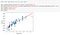Figure 86: Plotting the test data on the scatter plot with the regression line.

17. Plot the training data with the regression line:

18. Plot the complete data with regression line: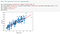Figure 88: Plotting the data on the scatterplot with the regression line.

19. Create a data frame for actual and predicted values:

19. Plot the bar graph for actual and predicted values:

20. Residual Sum of Square:

21. Calculating error:

So, that is how we can perform Simple Linear Regression from scratch with Python. Although Python libraries can perform all these calculations without diving in-depth, it is always good practice to know how these libraries perform such mathematical calculations.

Next, we will use the Scikit-learn library in Python to find the linear-best-fit regression line on the same data set. In the following code, we will see a straightforward way to calculate a simple linear regression using Scikit-learn.

# Simple Linear Regression Using Scikit-learn:

1. Import the required libraries:

3. Feature selection for regression model:

4. Plotting the data points on a scatter plot:

5. Dividing data into testing and training dataset:

6. Training the model:

7. Predicting values for a complete dataset:

8. Predicting values for training data:

9. Predicting values for testing data:

10. Plotting regression line for complete data: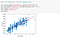Figure 102: Plotting the data on the scatter plot with a regression line.

11. Plotting regression line with training data: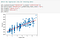Figure 103: Plotting the training data on a scatter plot with a regression line.

12. Plotting regression line with testing data: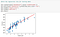Figure 104: Plotting the testing data on our scatter plot with a regression line.

13. Create dataframe for actual and predicted data points:

14. Plotting the bar graph for actual and predicted values: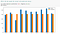Figure 106: Plotting a bar graph for actual vs. predicted values.

15. Calculating error in prediction:

Here we can see that we got the same output even if we use the Scikit-learn library. Therefore we can be sure that all the calculations we performed and derivations we understood are precisely accurate.

Please note that there are other methods to calculate the prediction error, and we will try to cover them in our future tutorials.

That is all for this tutorial. We hope you enjoyed it and learned something new from it. If you have any feedback, please leave us a comment or send us an email directly. Thank you for reading!

DISCLAIMER: The views expressed in this article are those of the author(s) and do not represent the views of Carnegie Mellon University. These writings do not intend to be final products, yet rather a reflection of current thinking, along with being a catalyst for discussion and improvement.

Published via Towards AI

# References:

 What is simple linear regression, Penn State, https://online.stat.psu.edu/stat462/node/91/

 scikit-learn, Getting Started, https://scikit-learn.org/

 SpongeBob SquarePants, Wikipedia, https://en.wikipedia.org/wiki/SpongeBob_SquarePants

 Deterministic: Definition and Examples, Statistics How To, https://www.statisticshowto.com/deterministic/

Written by

Written by

## Towards AI Team

#### Publishing the Best of Tech, Science, and Engineering | Editorial → https://towardsai.net/editorial | Subscribe→ https://towardsai.net/subscribe — @Towards_AI